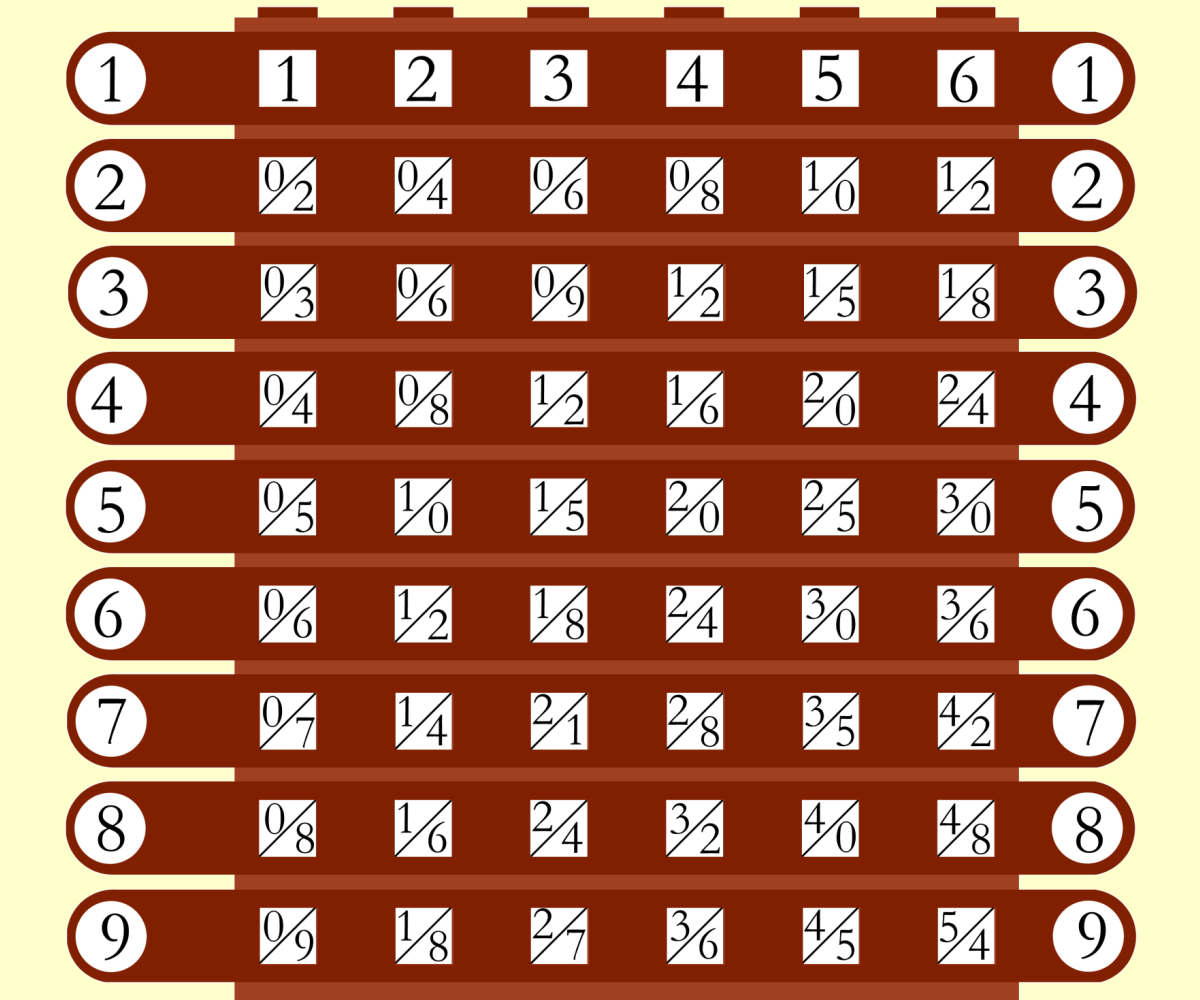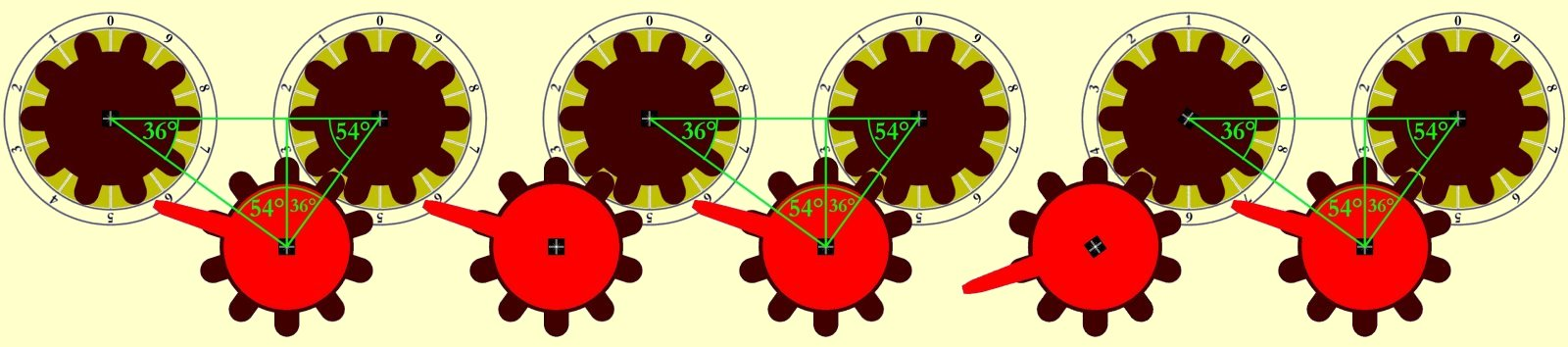[Computing] [Mechanical Calculators] [Schickard 1623]# The Schickard Calculator from 1623

The first digital calculating machine was invented in 1623 by Wilhelm Schickard (1592-1635), a professor at the university in Tübingen, Württemberg.

In 1624 a second model of the calculator was designed for Schickard's friend Johannes Kepler, but unfortunately was destroyed by a fire before completion.

After Wilhelm Schickard died of the plague during the Thirty Years' War, he and his invention fell into oblivion.
Therefore Blaise Pascal was credited with inventing the first mechanical calculator for a very long time.

In the nineteen-thirties two letters of Wilhelm Schickard were rediscovered in the estate of Johannes Kepler, where Schickard described his calculating machine in detail with all its parts.
As well two drawings of the machine were found and additionally construction instructions that Schickard had written to his mechanic Johann Pfister, who executed the construction of the machine.

On the basis of these documents, Bruno von Freytag-Löringhoff was able to reconstruct the mechanism and build a fully functional replica between 1957 and 1960.

## Components of the calculator

The Schickard Calculator consists of following parts:
• Multiplier
• Buffer Memory

## MultiplierThe multiplier consists of six turnable cylinders with ten sides each. Every side represents one digit from zero to nine.
There are nine rows on each side of the cylinders, representing the multiplication of the digit with the numbers from one to nine.

Shift registers are used to uncover the needed results of the multiplication tables and to cover those that are not needed.

The multiplier and multiplication using the machine are explained in the video "Multiplication with the Schickard Calculator".
There it is also explained how to multiply with Napier's bones, because they are the basis of multiplication with the Schickard machine.

Division is calculated as a reverse multiplication. This is explained in the video "Division with the Schickard Calculator".The adder is the core part of the Schickard Calculator, because it is needed for all four basic arithmetic operations.
The adder is by far the most complex part of the machine. It consists of following parts:

• six drums for the representation of the decimal places, each with the digits from zero to nine
• six viewing windows on the upper side of the adder to visualize the entered number
• six adjustment wheels for incrementing and decrementing the digits
• 11 gear wheels with ten teeth each (six wheels for the representation of the decimal places
and additionally five wheels for the rectification of the direction of rotation of the main wheels)
• five single tooth wheels for the tens-carry into the next higher decimal place
• and possibly a rasterization to prevent the wheels from blocking and overwinding

To ensure that the adder works properly, it is necessary that all gear wheels are arranged in clearly definied angles to each other.
The angle of 36 degrees is one-tenth of 360 degrees, which is directly derived from the ten-teeth gear wheels.
It is also important, that the angle between two main wheels and one auxiliary wheel is a right angle.
The third angle of 54 degrees results from the sum of angles of 180 degrees.

The inner construction of the adder and its usage for addition and subtraction are explained in the video Addition and subtraction with the Schickard calculator.

## Buffer Memory

The buffer memory is used to temporarily store a six-digit number. This is needed for all basic arithmetic operations, for example to store an intermediate result.

The buffer memory has a very simple construction. It consists of six turnable discs, each labled with the digits from zero to nine, where only the current digit is visible in a viewing window.

# Videos

The following videos explain design and usage of the Schickard Calculator using a replica of the machine.
The first video as well gives an overview over Schickard's extensive life's work and fields of activity.

### Wilhelm Schickard - Inventor of the first mechanical calculator

The first mechanical calculator was invented in 1623 by Wilhelm Schickard (1592-1635), a professor at the university in Tübingen, Württemberg. This video shows the three components of the machine (multiplier, adder and buffer memory). It as well gives an overview over Schickard's extensive life's work and fields of activity.

### Addition and Subtraction with the Schickard Calculator from 1623

This video shows the internal design and construction of the adder unit. Additionally addition and subtraction are shown in action using a replica of the machine.

### Multiplikation with the Schickard Calculator from 1623 and with Napier's Bones

This video shows multiplication with Schickard's calculator compared to multiplication with Napier's Bones, which were invented six years earlier by Scottish scholar John Napier.

### Division with the Schickard Calculator from 1623

With the first mechanical calculator, which was invented by Wilhelm Schickard in 1623, all four basic arithmetic operations could be performed. This video shows division with the Schickard calculator. At first there is an easy example for the basic understanding. It is then shown that even with this nearly 400 year old calculator floating point divisions could be performed.

### Calculating the Square Root of 13with the Schickard Calculator from 1623

Not only the four basic arithmetic operations were supported by the Schickard Calculator, but also the calculation of square roots. This video shows how that was performed by calculating the square root of 13 as an example.

### Calculation of lunar orbits per solar yearusing the Schickard Calculator from 1623

In this video the number of lunar orbits during one solar year is calculated using the Schickard Calculator from 1623. It is ancient knowledge that there are 254 lunar orbits in 19 solar years, therefore the number of lunar orbits in one solar year equals to 254 divided by 19.

## Literature

• "Wilhelm Schickard 1592 - 1635 · Astronom, Geograph, Orientalist, Erfinder der Rechenmaschine"